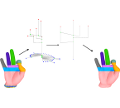## Reeb graphs.Harish Doraiswamy and Vijay Natarajan.
Poster at Microsoft TechVista, Chennai, 2008

### Abstract

Isosurface or level sets are used extensively to visualize three and higher dimensional scientific data. The Reeb graph tracks topology changes in level sets of a scalar function, and therefore serves as a useful user interface for selecting meaningful level sets. Besides visualization, Reeb graphs also find applications in geometric modeling and shape matching. We describe two algorithms for constructing the Reeb graph of a smooth function defined over manifolds in any dimension. The first algorithm maintains connected components of level sets as a dynamic graph and constructs the Reeb graph in O(n log(n) + n log(g)(log log(g))3) time for three-dimensional input, where n is the number of triangles in the tetrahedral mesh representing the input volume and g is the maximum genus over all level sets of the function. Our algorithm extends to higher dimensions where we construct Reeb graphs in O(n log(n) (log log(n))3) time. This is a significant improvement over the previously known O(n2) algorithm.

In a complementary approach, we design a near-optimal two step algorithm that is simple and easy to implement. This algorithm identifies critical points of the input function in the first step, and connects the critical points in the second step to obtain the Reeb graph. Experimental results show that our two-step algorithm is an order of magnitude faster than existing methods. We also develop methods to simplify the Reeb graph, which aids in removing noise and unimportant features from the input, and produce a feature-directed layout of the Reeb graph, which helps users explore their data effectively.